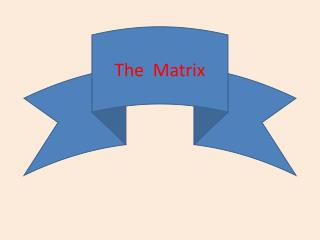DownloadDownload PresentationThe Matrix

# The Matrix

Télécharger la présentation## The Matrix

- - - - - - - - - - - - - - - - - - - - - - - - - - - E N D - - - - - - - - - - - - - - - - - - - - - - - - - - -
##### Presentation Transcript

1. The Matrix

2. Matrices Exercises 1- Three companies X,Y,F two types of fabrics ,X company have produced a 1000 meter from the first type , 1200 from the second type. Y company have produced a 500 meters from the first type . 900 meters from the second type , and F have produced a 700 meter from the first type, 400 meters from the second type _ Write these data in matrix image ( A ) on 3 x 2 system _ Write these data also in matrix image ( B ) on 2 x 3 system

3. 2- Two clothing shops one of those days the first shop sell 20 shirts. 5 suits, 12 shoes, and the second shop have sell 13 shirts, 3 suits, 14 shoes _ Write these data in matrix image ( X ) on 2 x 3 system

4. 3- if the matrix was -2 3 5 -6 2 A.= 6 4 , B = 8 5 4 6 0 complete the following -i- system A is ………… , system B is ………… ( ii ) B12 = …………….. iii - Element A23 = …………….. , Element B31 is ……………….. , A13 + B31 = ……………………. 4- Write the matrix A on 2 x 3 system , write matrix B on 2 x 2 system

5. Rounded matrix and the equal of two matrixes : X2 -3 4 -3 5- If it was= 5 Y+2 0 8 Then find the values of X,Y 6 X 5 6 1 5 6-If it was = Y+X 4 7 5 4 F Then find the values of X,Y ,F

6. 7- Prove that the Equality of X and Y values cannot be achieved for the following: 3 X – Y 7 5 7 = 2 Y 1 -4 X – Y 4 -3 4 -3 6 8-if it was A = , B = 0 5 0 8 9 - Mention the systems of A,B and then find AMD ,BMD

7. 2 X 2 Y 9- if A was= , B = -1 5 4 5 And BMD then find X,Y 10 – complete : The Matrix is a particular organization of data on the picture ........ Horizontal and………. vertical placed between.......... Matrix systems is ..............

8. 2 1 -1 3 11- If A was= -3 2 , B= 0 1 5 1 7 -4 Then find A + B , 2A – 3 B 3 -2 5 -2 3 1 12-If A was = 1 4 0 . B= 2 -3 5 2 -3 1 0 4 7 Then find each of : A + BMD , AMD – B . AMD+BMD if it possible…

9. 3 3 1 3 4 -2 14-If X was= .Y= 5 -1 2 5 1 0 Find the matrix A that achieve the relevant : : 2 A + Y – X = 3 -2 7 3 15 – if Y + 2 YMD = 1 4 6 1 Then find the matrix X

10. Multiplication 3 2 -2 1 16-If A was= , B= 4 1 3 4 Then find A B ,, B A 0 -2 3 1 5 17- if X was= .Y= 1 4 1 4 5 4 0 Find (X Y )MD , X – Y 2

11. -2 1 3 0 18- if A was= , B= 4 2 4 1 Then find the matrix X so 2 X + XMD = A B 3 -2 19- if A was= 4 -3 - prove that A2 - 4A + 4 I = ( A – 2 I )2

12. 2 1 20- if A was = 3 1 - prove that A2 - A – 5 I = 1 -2 21 – If X = then find X4 0 2 0 -2 3 1 2 1 22- If A was= 1 4 1 ,B= 1 0 2 4 1 -1 2 5 1 Then find A2 + 2 AB + B2

13. With MY BEST WISHES With MY BEST WISHES With MY BEST WISHES With MY BEST WISHES

14. #أسماء الطالبات: الهنوفالسديس أمجاد المطيري أفنان القحطاني هيا الماضي ود القاسم الهنوفالتويجري O3#الشعبة: